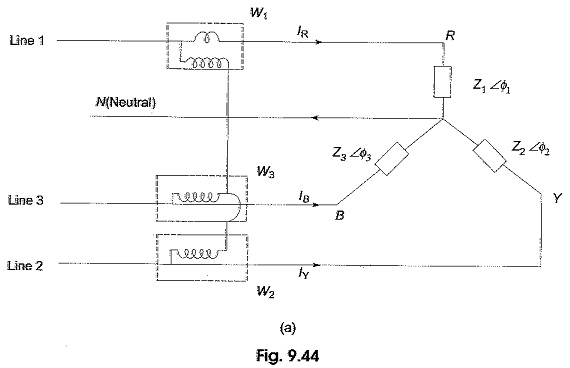## Power in Three Phase Circuits:

Measurement of power by a wattmeter in a single phase circuit can be extended to measure Power in Three Phase Circuits. From earlier Section, it is clear that we require three wattmeters, one in each phase to measure the power consumed in a three-phase system.

Obviously, the total power is the algebraic sum of the readings of the three wattmeters. In this way we can measure power in balanced and unbalanced loads.

In a balanced case it would be necessary to measure power only in one phase and the reading is multiplied by three to get the total power in all the three phases. This is true in principle, but presents a few difficulties in practice. To verify this fact let us examine the circuit diagram in Fig. 9.44(a) and (b).Observation of Figs 9.44(a) and (b) reveals that for a star-connected load, the neutral must be available for connecting the pressure coil terminals. The current coils must be inserted in each phase for a delta-connected load. Such connections sometimes may not be practicable, because the neutral terminal is not available all the time in a star-connected load, and the phases of the delta-connected load are not accessible for connecting the current coils of the wattmeter.

In most of the commercially available practical three-phase loads, only three line terminals are available. We, therefore, require a method where we can measure Power in Three Phase Circuits with an access to the three lines connecting the source to the load.

Scroll to Top